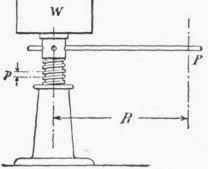The ordinary jack screw is a good example of the wedge principle. It is a screw in combination with a lever.

Figure 31 shows a common jack screw. The thread is the inclined plane or wedge and the circumference of the screw or thread corresponds to the base of the plane. The force P on the handle is the force acting parallel to the base and the weight W is the weight lifted. The same rule which is used for the wedge will now apply. If the length of the handle, the pitch of the thread (the distance between two successive threads), and the force applied to the handle are known, the weight which can be lifted, neglecting friction, can easily be calculated.

Referring to Fig. 31, for every turn of the handle the weight is raised an amount equal to the pitch:

P X C = W X p where P, W, and p are as shown in the sketch and C is the circular distance the end of the handle moves through in making one turn, or

C = 2 π RFig. 30. - Helix.Fig. 31. - Jack Screw.